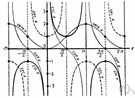# trigonometric function

(redirected from Sin-cos-tan)
Also found in: Thesaurus, Encyclopedia.
trigonometric function
In a right triangle, the three main trigonometric functions are
sine θ = opposite / hypotenuse
cosine θ = adjacent / hypotenuse
tangent θ = opposite / adjacent.

## trigonometric function

n.
A function of an angle expressed as the ratio of two of the sides of a right triangle that contains that angle; the sine, cosine, tangent, cotangent, secant, or cosecant. Also called circular function.

## trigonometric function

n
1. (Mathematics) Also called: circular function any of a group of functions of an angle expressed as a ratio of two of the sides of a right-angled triangle containing the angle. The group includes sine, cosine, tangent, secant, cosecant, and cotangent
2. (Mathematics) any function containing only sines, cosines, etc, and constants

## trig′onomet′ric func′tion

n.
a function of an angle, as the sine or cosine, expressed as the ratio of the sides of a right triangle.
Also called circular function.
[1905–10]
trigonometric function
In a right triangle, the trigonometric functions are: sine θ = opposite/hypothenuse cosine θ = adjacent/hypothenuse tangent θ = opposite/adjacent

## trig·o·no·met·ric function

(trĭg′ə-nə-mĕt′rĭk)
A function of an angle, as the sine, cosine, or tangent, whose value is expressed as a ratio of two of the sides of the right triangle that contains the angle.
ThesaurusAntonymsRelated WordsSynonymsLegend:
 Noun 1trigonometric function - function of an angle expressed as a ratio of the length of the sides of right-angled triangle containing the anglecircular functionfunction, mapping, mathematical function, single-valued function, map - (mathematics) a mathematical relation such that each element of a given set (the domain of the function) is associated with an element of another set (the range of the function)sine, sin - ratio of the length of the side opposite the given angle to the length of the hypotenuse of a right-angled trianglearc sine, arcsin, arcsine, inverse sine - the inverse function of the sine; the angle that has a sine equal to a given numbercos, cosine - ratio of the adjacent side to the hypotenuse of a right-angled trianglearc cosine, arccos, arccosine, inverse cosine - the inverse function of the cosine; the angle that has a cosine equal to a given numbertangent, tan - ratio of the opposite to the adjacent side of a right-angled trianglearc tangent, arctan, arctangent, inverse tangent - the inverse function of the tangent; the angle that has a tangent equal to a given numbercotan, cotangent - ratio of the adjacent to the opposite side of a right-angled trianglearc cotangent, arccotangent, inverse cotangent - the inverse function of the cotangent; the angle that has a cotangent equal to a given numbersec, secant - ratio of the hypotenuse to the adjacent side of a right-angled trianglearc secant, arcsec, arcsecant, inverse secant - the inverse function of the secant; the angle that has a secant equal to a given numbercosec, cosecant - ratio of the hypotenuse to the opposite side of a right-angled trianglearc cosecant, arccosecant, inverse cosecant - the angle that has a cosecant equal to a given number
Site: Follow: Share:
Open / Close